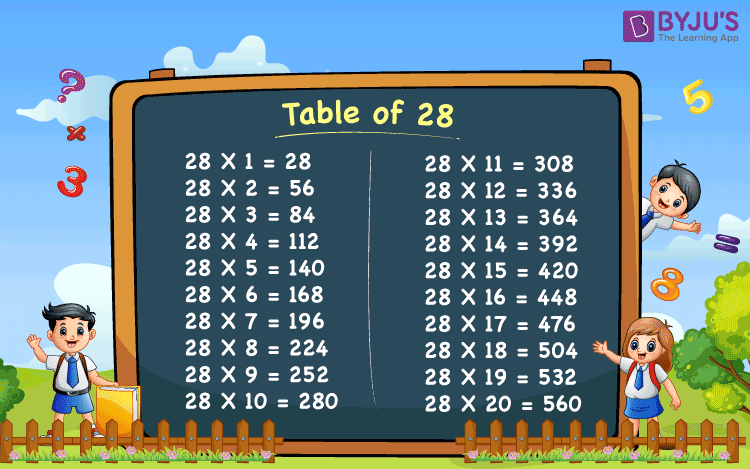# Table of 28

In this, we will read and memorize the table of 28. You can also download the 28 times table image from here for further reference. Multiplication tables help us to solve mathematical problems easily and quickly. Let’s look at the table here.

## Table of 28 Chart## 28 Times Table

Tables from 1 to 20 are important to study the further numbers tables.

 28 X 1 = 28 28 X 2 = 56 28 X 3 = 84 28 X 4 = 112 28 X 5 = 140 28 X 6 = 168 28 X 7 = 196 28 X 8 = 224 28 X 9 = 252 28 X 10 = 280 28 X 11 = 308 28 X 12 = 336 28 X 13 = 364 28 X 14 = 392 28 X 15 = 420 28 X 16 = 448 28 X 17 = 476 28 X 18 = 504 28 X 19 = 532 28 X 20 = 560

### Examples of Table of 28

Repeatedly adding by 28’s gives the table of 28.

(i) When 2 packs each having 28 pastel colours.

By repeated addition we can show 28 + 28 = 56

Then, twenty-eight times 2 is

2 × 28 = 56

Therefore, there are 56 pastel colours.

(ii) When 3 bunches of 28 bananas each.

By repeated addition we can show 28 + 28 + 28 =84 Then, twenty-eight times 3 is

3 × 28 = 84

Therefore, there are 84bananas.

(iii) When 4 bunches of 28 bananas each.

By repeated addition we can show 28 + 28 + 28 +28 = 112

Then, twenty-eight times 4 is

4 × 28 = 112

Therefore, there are 112 bananas.

(iv) When 5 bunches of 28 bananas each.

By repeated addition we can show 28 + 28 + 28 + 28 +28 = 140

Then, twenty-eight times 5 is

5 × 28 = 140

Therefore, there are 140 bananas.

(v) When 6 bunches of 28 bananas each.

By repeated addition we can show 28 + 28 + 28 + 28 + 28 + 28 = 168

Then, twenty-eight times 6 is

6 × 28 = 168

Therefore, there are 168 bananas.

(vi)When 7 bunches of 28 bananas each.

By repeated addition we can show 28 + 28 + 28 + 28 + 28 + 28 +28 = 196

Then, twenty-eight times 7 is

7 × 28 = 196

Therefore, there are 196 bananas.

(vii) When 8 bunches of 28 bananas each.

By repeated addition we can show 28 + 28 + 28 + 28 + 28 + 28 + 28 + 28 = 224

Then, twenty-eight times 8 is

8 × 28 = 224

Therefore, there are 224 bananas.

(viii) When 9 bunches of 28 bananas each.

By repeated addition we can show 28 + 28 + 28 + 28 + 28 + 28 + 28 + 28 +28 = 252

Then, twenty-eight times 9 is

9 × 28 = 252

Therefore, there are 252 bananas.

(ix) When 10 bunches of 28 bananas each.

By repeated addition we can show 28 + 28 + 28 + 28 + 28 + 28 + 28 + 28 +28 +28 = 280

Then, twenty-eight times 10 is

10 × 28 = 280

Therefore, there are 280 bananas.

Register to BYJU’S to get all the tables from 1 to 30.

 Explore more multiplication Tables Table of 12 Table of 13 Table of 15 Table of 16 Table of 17 Table of 18 Table of 19 Table of 20
Test your Knowledge on Table of 28